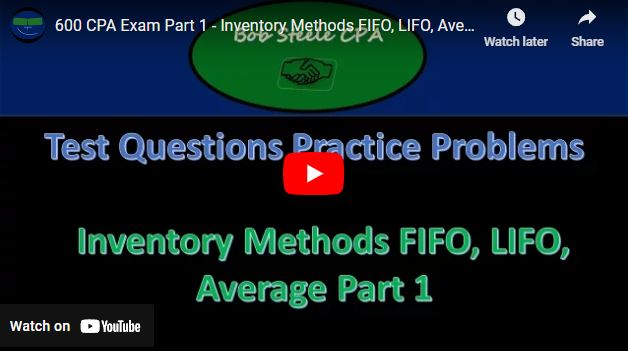## 600 CPA Exam Part 1 – Inventory Methods FIFO, LIFO, Average – CPA exam & other accounting test prep

In this blog post, we will delve into the world of accounting, specifically inventory accounting. We will work through several practice problems to understand how to calculate the per unit value of ending inventory, using both the weighted average perpetual inventory system and the FIFO (First-In-First-Out) perpetual inventory method. We’ll also explore how to adjust inventory figures when errors occur. So, let’s dive right in!

Problem 1: Weighted Average Perpetual Inventory System

Imagine you’re working with a company that uses a weighted average perpetual inventory system. They’ve provided you with data on purchases, sales, and prices. Your task is to determine the per unit value of ending inventory as of August 31.

Solution:

In this case, we’ll create a table with six columns to keep track of our calculations. The columns will represent sales, purchases, units, cost per unit, total cost, and running totals. We’ll use this format to solve the problem step by step.

1. Initial Purchase:
• Units: 9
• Cost per unit: \$11
• Total cost: 9 * \$11 = \$99
2. Second Purchase:
• Units: 11
• Cost per unit: \$15
• Total cost: 11 * \$15 = \$165
3. Average Calculation:
• Total units: 9 + 11 = 20
• Total cost: \$99 + \$165 = \$264
• Average cost per unit: \$264 / 20 = \$13.20
4. Sale:
• Units sold: 18
• Cost per unit (average): \$13.20
• Cost of sales: 18 * \$13.20 = \$237.60
5. Ending Inventory:
• Remaining units: 20 – 18 = 2
• Cost per unit (average): \$13.20
• Value of ending inventory: 2 * \$13.20 = \$26.40
• Units: 14
• Cost per unit: \$14
• Total cost: 14 * \$14 = \$196
7. New Average Calculation:
• Total units: 20 (previous) + 14 (new) = 34
• Total cost: \$264 (previous) + \$196 (new) = \$460
• New average cost per unit: \$460 / 34 ≈ \$13.53

Now you’ve successfully determined the per unit value of ending inventory using the weighted average perpetual inventory system.

Problem 2: FIFO Perpetual Inventory Method

Now, let’s explore the FIFO perpetual inventory method to find the value of ending inventory on August 15. We’ll use a similar six-column format for this problem.

Solution:

1. Initial Inventory:
• Units: 32
• Cost per unit: \$22
• Total cost: 32 * \$22 = \$704
2. First Purchase:
• Units: 27
• Cost per unit: \$21
• Total cost: 27 * \$21 = \$567
3. Second Purchase:
• Units: 31
• Cost per unit: \$22
• Total cost: 31 * \$22 = \$682
4. Sale:
• Units sold: 64
• Cost per unit (FIFO): First, use the oldest units, which are the initial 32 at \$22.
• Cost of sales: 32 * \$22 = \$704
5. Remaining Units after Sale:
• 64 (total sold) – 32 (initial) = 32 units
• Cost per unit (FIFO): Now use the next oldest units, 27 at \$21.
• Cost of remaining inventory: 32 * \$21 = \$672
6. Ending Inventory:
• Remaining units: 31 (from the most recent purchase)
• Cost per unit (FIFO): \$22
• Value of ending inventory: 31 * \$22 = \$682

So, using the FIFO perpetual inventory method, the value of ending inventory on August 15 is \$682.

Problem 3: Correcting Inventory Figures

Suppose a company’s income statement includes sales, cost of goods sold, and gross profit. It’s known that the beginning inventory figure is correct, but the ending inventory was overstated by \$24,000. You need to determine the correct gross profit.

Solution:

In this case, we need to recalculate the cost of goods sold (COGS) using the corrected ending inventory figure.

1. Beginning Inventory: \$130,800
2. Purchases: \$277,000
3. Total Available Inventory: \$130,800 + \$277,000 = \$407,800

Now, we need to adjust the ending inventory by subtracting the overstatement:

• Corrected Ending Inventory = \$140,800 (original) – \$24,000 (overstatement) = \$116,800

Next, calculate the correct COGS:

• Correct COGS = Total Available Inventory – Corrected Ending Inventory
• Correct COGS = \$407,800 – \$116,800 = \$291,000

Finally, calculate the correct gross profit:

• Correct Gross Profit = Sales – Correct COGS
• Correct Gross Profit = \$141,000 – \$291,000 = -\$150,000

So, the correct gross profit is -\$150,000 after adjusting for the overstatement in the ending inventory.

Conclusion: Inventory accounting involves various methods and calculations to determine the value of ending inventory and calculate important financial figures like cost of goods sold and gross profit. By practicing problems like these, you can enhance your accounting skills and handle real-world inventory scenarios more effectively.